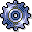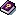Target / Actual ComparisonUse

Use for product drilldowns:

Report with which you can analyze the target and actual data in the following application components of Product Cost Controlling using product drilldown:

• Product Cost by Period
• Product Cost by Order

The reporting time frame can be cumulative or limited.

This report is used to compare the target and actual data that was determined duringVariance Calculation. The results of variance calculation are based on the target cost versions specified for the report: Different variances are considered, depending on the target cost version (also see:Types of Variance Calculation,Target Cost Versions in Variance Calculation).

Features

Data Displayed

The following data is shown based on key figures and/or original cost elements:

• Target costs
• Actual costs
• Target costs per unit
• Actual costs per unit
• Absolute variances = Actual costs – Target costs
• Percentage variances = Absolute variances / Target costs

The variances can also be displayed per unit.

The above figures are based on the costs per unit of output.

• Target quantity
• Confirmed quantity
• Absolute variances = Target quantity – Confirmed quantity
• Percentage variances = Absolute variances / Confirmed quantity

• Object information
• Reporting time frame

Integration

Prerequisites

Make sure that the variances have been calculated for the specific target cost version in the relevant reporting time frame. Reports of summarized analysis require that a data collection run be executed beforehand.

Notes

Recommendation

For more information on the production variances, use the target / actual / production variances - reports, or the reports on the variance catagories.

For Product Drilldown

If you would like to analyze target costs in summarized reports, note the information in Background Information on Summarized Analysis to ensure a proper interpretation of the data.